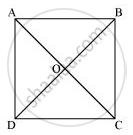# Show that the diagonals of a square are equal and bisect each other at right angles. - Mathematics

Show that the diagonals of a square are equal and bisect each other at right angles.

#### SolutionLet ABCD be a square. Let the diagonals AC and BD intersect each other at a point O. To prove that the diagonals of a square are equal and bisect each other at right angles, we have to prove AC = BD, OA = OC, OB = OD, and ∠AOB = 90º.

In ΔABC and ΔDCB,

AB = DC (Sides of a square are equal to each other)

∠ABC = ∠DCB (All interior angles are of 90°)

BC = CB (Common side)

∴ ΔABC ≅ ΔDCB (By SAS congruency)

∴ AC = DB (By CPCT)

Hence, the diagonals of a square are equal in length.

In ΔAOB and ΔCOD,

∠AOB = ∠COD (Vertically opposite angles)

∠ABO = ∠CDO (Alternate interior angles)

AB = CD (Sides of a square are always equal)

∴ ΔAOB ≅ ΔCOD (By AAS congruence rule)

∴ AO = CO and OB = OD (By CPCT)

Hence, the diagonals of a square bisect each other.

In ΔAOB and ΔCOB,

As we had proved that diagonals bisect each other, therefore,

AO = CO

AB = CB (Sides of a square are equal)

BO = BO (Common)

∴ ΔAOB ≅ ΔCOB (By SSS congruency)

∴ ∠AOB = ∠COB (By CPCT)

However, ∠AOB + ∠COB = 180º (Linear pair)

2∠AOB = 180º

∠AOB = 90º

Hence, the diagonals of a square bisect each other at right angles.

Concept: Another Condition for a Quadrilateral to Be a Parallelogram
Is there an error in this question or solution?
Chapter 8: Quadrilaterals - Exercise 8.1 [Page 146]

#### APPEARS IN

NCERT Class 9 Maths